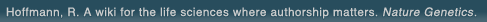# The world's first wiki where authorship really matters (Nature Genetics, 2008). Due credit and reputation for authors. Imagine a global collaborative knowledge base for original thoughts. Search thousands of articles and collaborate with scientists around the globe.

wikigene or wiki gene protein drug chemical gene disease author authorship tracking collaborative publishing evolutionary knowledge reputation system wiki2.0 global collaboration genes proteins drugs chemicals diseases compound
Hoffmann, R. A wiki for the life sciences where authorship matters. Nature Genetics (2008)# Solution thermodynamics of alkyl p-aminobenzoates.

Equilibrium solubilities of the first four homologous alkyl p-aminobenzoate esters were determined in methanol, ethanol, and 1-propanol at 25, 33, and 40 degrees; the esters and the alcohols comprise separate homologous series. The solution process of a solute may be considered to be the summation of two sequential steps, melting and mixing, and the magnitude of solubility depends upon temperature and the extent of interactions between solute and solvent molecules. Quantitative solute concentrations, obtained from spectrophotometric analysis, were converted to mole fractions. Statistical analysis of the logarithmic mole fraction solubilities of the aminobenzoates, which were linear with respect to both reciprocal absolute temperature and the logarithm of absolute temperature, generated enthalpies and entropies of solution, respectively. The heats of fusion and the melting points of these aminobenzoates were determined to calculate their ideal solubilities. Excess free energies and partial molal free energies of each solution were calculated from the activity coefficients of the solutes; the thermodynamic elements for these systems are discussed.

## References

1. Solution thermodynamics of alkyl p-aminobenzoates. Schwartz, P.A., Paruta, A.N. Journal of pharmaceutical sciences. (1976) [Pubmed]